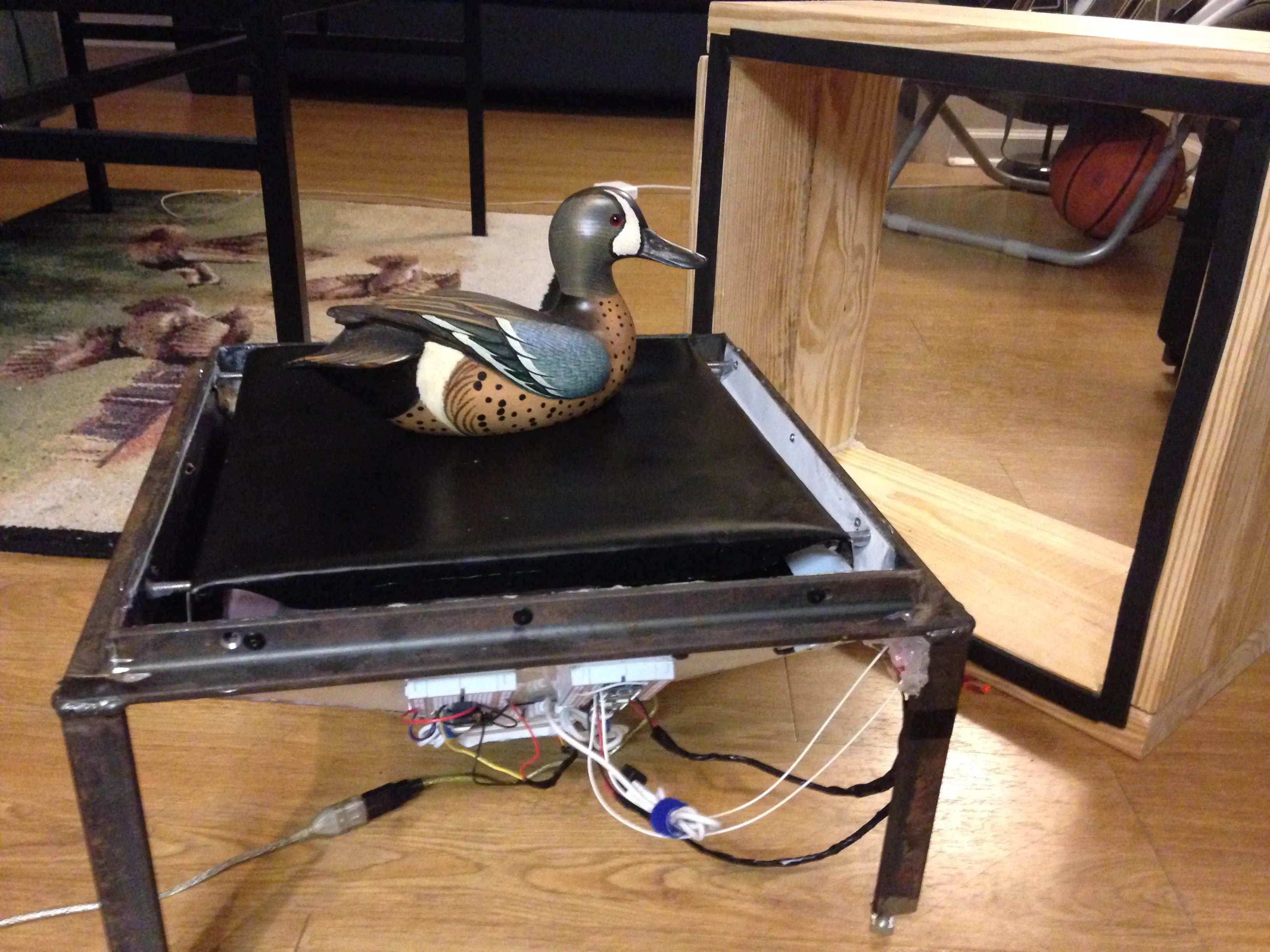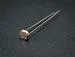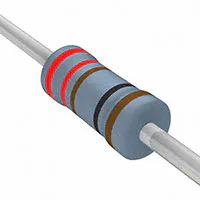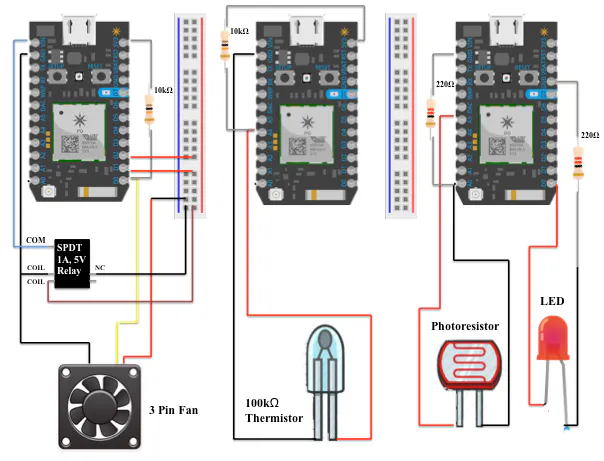# Carbon Fiber Vacuum Chamber

Our project is a carbon fiber vacuum chamber that is monitored by multiple particle photons and various sensors.

IntermediateProtipOver 1 day4,385## Things used in this project

### Hardware components

 3 Pin Fan
×1
 100k Thermistor
×1LED (generic) Included with particle
×1Photo resistor Included with particle
×1
 Relay (generic) SPDT 1A, 5V Relay
×1Resistor 10k ohm
×2Resistor 221 ohm
×2Particle Photon
×3

### Software apps and online services

 Particle
 Particle.io

## Custom parts and enclosures

### CREO Files for project

Here are the creo files for the hard components of the project.

## Schematics

### Circuit Diagram

Wire the components accordingly## Code

### Fan

C/C++
The fan code triggers fan on and records fan speed
```int counter = D0; //yellow wire from 3 pin fan
int period;
int frequency;
int rpm;
int power = D1;
int power2 = D2;

void setup()
{

pinMode(counter,INPUT_PULLDOWN); // counter needs 3.3v pulldown
pinMode(power, OUTPUT); // 5v relay needs 2 sources to power it on. power and power2
pinMode(power2, OUTPUT);
Particle.subscribe("tjohn212LidStatus", myHandler); // using the unique name from lidsensor, subscribe to the event. Assign myHandler code below
delay(2000);

}

void loop()
{

period = (pulseIn(D0, HIGH))/3600; // the PWM provides a period, or duration between pulses
frequency = (10 / period); // must take the inverse of period to get frequency
rpm = (frequency*60); // and finally convert frequency to RPM
Particle.publish("rpm", String(rpm)); // publish final value to console
delay(1000);
}

void myHandler(const char *event, const char *data) // here is were you assign myHandler task
{

if (strcmp(data,"ON")==0) // we have to convert the incoming data using strcmp() which compares two chars.
{
digitalWrite(power,HIGH); // if the lid is on from the other photon, turn fan on
digitalWrite(power2,HIGH);
delay(500);
}
else if (strcmp(data,"OFF")==0)
{
digitalWrite(power,LOW); // if the lid is off turn fan off
digitalWrite(power2,LOW);
delay(500);
}
}
```

### Lid Sensor

C/C++
The lid code turns on LED based on whether or not the lid is on or not
```int lidon;
int lidoff;
int led = D0; // Where LED is plugged into. The other side goes to a resistor connected to GND.
int photoresistor = A0; // Where photoresistor is plugged into. The other side goes to the "power" pin (below).
int power = A5; // Other end of your photoresistor. The other side is plugged into the "photoresistor" pin (above).
int analogvalue; // Here we are declaring the integer variable analogvalue, which we will use later to store the value of the photoresistor.
bool lid = false;

void setup()
{

pinMode(led,OUTPUT); // LED pin is output
pinMode(photoresistor,INPUT);  // input reading from photoresistor
pinMode(power,OUTPUT); // pin powering photoresistor
digitalWrite(power,HIGH); // write the photoresistor to max power
}

void loop()
{

delay(10);
if (analogvalue < 5) //if value above is less than, turn ON LED
{
digitalWrite(led,HIGH);
if (lid==true)
{
Particle.publish("tjohn212LidStatus","ON"); // using unique event name, publish to console so other photon can subscribe
delay(1000);
lid=false;
}
}
else
{
digitalWrite(led,LOW); // any other value not less than, turn OFF LED
if (lid==false)
{
Particle.publish("tjohn212LidStatus","OFF");
delay(1000);
lid=true;
}
}
}
```

### Thermistor

C/C++
Thermistor code publishes temperature readings to console
```#include <math.h>
const int pullup_res = 10000; // ( 10kOm )
const double beta = 4390; // in K for Semitec 104 GTA-2
const double thermistor_res = 100000; // 100kOm thermistor
const double thermistor_nom_temp = 77; // Nominal Farenheit

void setup()
{
}

void loop()
{
delay(1000);
}

void thermistor_temp(int aval)
{
double R, T;
R = (double) pullup_res / ( (4095 / (double) aval ) - 1 );

T = 1 / ( ( 1 / (thermistor_nom_temp + 241.15 )) + ( ( 1 / beta) * log ( R / thermistor_res ) ) );

T -= 241.15; // convert K to F

// return degrees F
Particle.publish("Temperature", String(T) + " °F"); // publishes temp to console every 2 seconds
delay(2000);
}
```

## Credits

### Tommy Scheetz

1 project • 2 followers

### Dylan Ramsey

1 project • 2 followers